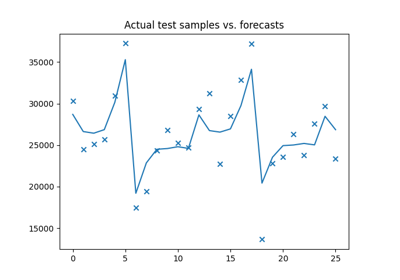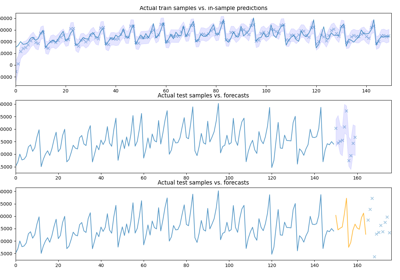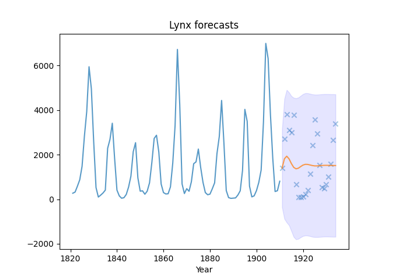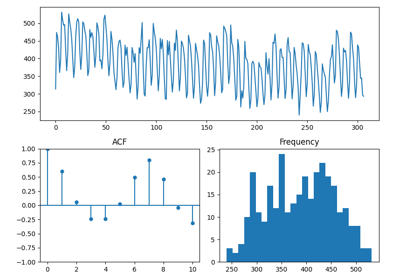# pmdarima.model_selection.train_test_split¶

pmdarima.model_selection.train_test_split(*arrays, test_size=None, train_size=None)[source][source]

Split arrays or matrices into sequential train and test subsets

Creates train/test splits over endogenous arrays an optional exogenous arrays. This is a wrapper of scikit-learn’s train_test_split that does not shuffle.

Parameters: *arrays : sequence of indexables with same length / shape Allowed inputs are lists, numpy arrays, scipy-sparse matrices or pandas dataframes. test_size : float, int or None, optional (default=None) If float, should be between 0.0 and 1.0 and represent the proportion of the dataset to include in the test split. If int, represents the absolute number of test samples. If None, the value is set to the complement of the train size. If train_size is also None, it will be set to 0.25. train_size : float, int, or None, (default=None) If float, should be between 0.0 and 1.0 and represent the proportion of the dataset to include in the train split. If int, represents the absolute number of train samples. If None, the value is automatically set to the complement of the test size. splitting : list, length=2 * len(arrays) List containing train-test split of inputs.

Examples

>>> import pmdarima as pm
>>> from pmdarima.model_selection import train_test_split
>>> y_train, y_test = train_test_split(y, test_size=50)
>>> y_test.shape
(50,)


The split is sequential:

>>> import numpy as np
>>> from numpy.testing import assert_array_equal
>>> assert_array_equal(y, np.concatenate([y_train, y_test]))


## Examples using pmdarima.model_selection.train_test_split¶Simple auto_arima modelPipelines with auto_arimaPersisting an ARIMA modelFitting an auto_arima model